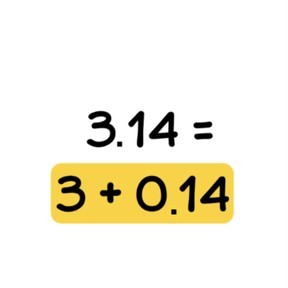Place value - decimal numbers with 1 or 2 decimal places

# Place value - decimal numbers with 1 or 2 decimal places

Place value - decimal numbers with 1 or 2 decimal places8,000 schools use Gynzy92,000 teachers use Gynzy1,600,000 students use Gynzy

## General

Students learn the structure of decimal numbers with 1 or 2 decimal places and they learn the value of digits in a number as well as how to place decimal numbers into a chart.

4.NF.C.5
4.NF.C.6

## Relevance

It is important to know how a decimal number is put together, so students can learn to calculate with them.

## Introduction

Start with a quiz about decimal numbers. Discuss with decimal places with the students and make sure that you mention how to say the decimal numbers out loud.

## Development

Discuss what tenths and hundredths are with your students. A tenth is written as 0.1 and fits 10 times into 1.0. A hundredth is written as 0.01 and it fits 100 times into 1.0. Check that students understand this by asking questions. How do you say 0.7 and 0.05? Show the different parts of a decimal number and use a decimal place value chart. Show, using steps on a number line, how you can divide a decimal number into different parts. The decimal number of 12.53 can be split into 10.00 + 2.00 + 0.50 + 0.03. Discuss the value of the digits in the decimal number: The 1 represents 1 tens, and has the value of 10.00. The 2 represents 2 ones and has a value of 2.00. The 5 represents 5 tenths and has a value of 0.50. The 3 represents 3 hundredths and has a value of 0.03. Practice setting decimal numbers into the decimal place value chart together with the students, how to read the decimal numbers using the decimal place value chart, and filling in the missing number in a decimal place value chart using the number line.

Check that students understand how to build decimal numbers with 1 or 2 decimals by asking the following questions:
- How many tenths does 4.9 have?
- Is the 3 a tenth or a hundredth in the number 2.3?
- What is 3.00 + 0.50 + 0.09?

## Guided practice

Students add ones and tens. You write the total. Together you fill in the missing numbers on the number line. You then fill in the gaps with missing decimal numbers.

## Closing

Discuss with students why it is important to know how decimal numbers are put together. If they know what the parts of a decimal number are, they can learn to do problems with decimal numbers. Finish with a quiz about decimal numbers. This time about the value of the digits in decimal numbers.

## Teaching tips

Decimal numbers can be very abstract for students. A part of a number, like 0.03, does not say much intrinsically. Make it visual by using money, scale, stopwatch, etc. Make sure that students are comfortable with this goal before moving to calculating with decimal numbers.

### The online teaching platform for interactive whiteboards and displays in schools

• Save time building lessons

• Manage the classroom more efficiently

• Increase student engagement